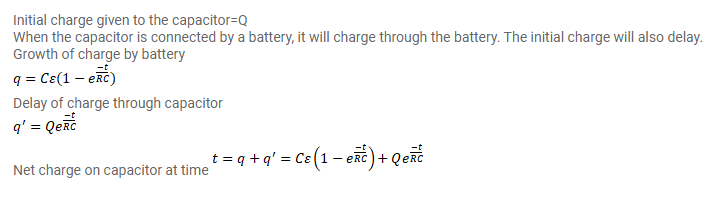# A capacitor of capacitance C is given a charge Q.

Question:

A capacitor of capacitance $\mathrm{C}$ is given a charge $\mathrm{Q} .$ At $t=0$, it is connected to an ideal battery of emf $\varepsilon$ through a resistance $\mathrm{R}$. Find the charge on capacitor at time $t$.

Solution: